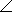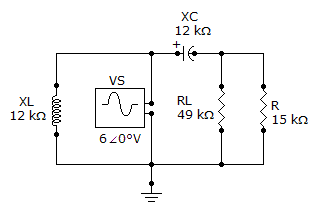# Online Electrical Engineering Test - Electrical Engineering Test 5Loading Test...

Instruction:

• This is a FREE online test. DO NOT pay money to anyone to attend this test.
• Total number of questions : 20.
• Time alloted : 30 minutes.
• Each question carry 1 mark, no negative marks.
• DO NOT refresh the page.
• All the best :-).

1.

How much resistance is required to limit the current from a 12 V battery to 3.6 mA?

A.
 3.3 kB.
 33 kC.
 2.2 kD.
 22 k2.

The ampere is the unit of resistance.

A.
 TrueB.
 False3.

When a fourth resistor is connected in series with three resistors, the total resistance

A.
 increases by one-fourthB.
 increasesC.
 decreasesD.
 remains the same4.

A 120load is connected across an ideal voltage source with VS = 12 V. The voltage across the load

A.
 is 0 VB.
 is 12 VC.
 is 120 VD.
 cannot be determined5.

What is the reluctance of the circuit in problem 9 if the flux is 275Wb?

A.
 0.0066 At/WbB.
 0.066 At/WbC.
 8,727 At/WbD.
 872 At/Wb6.

Hysteresis is the number of lines of force per unit area in a magnetic field.

A.
 TrueB.
 False7.

Electromagnetic induction is the force that produces a magnetic field.

A.
 TrueB.
 False8.

Reluctance in a magnetic circuit is analogous to current in an electrical circuit.

A.
 TrueB.
 False9.

If the rms current through a 4.7 kresistor is 4 mA, the peak voltage drop across the resistor is

A.
 4 VB.
 18.8 VC.
 26.6 VD.
 2.66 V10.

The mutual inductance when k = 0.65, L1 = 2H, and L2 = 5H is

A.
 2 mHB.
 2HC.
 4HD.
 8H11.

If the voltage is stepped up, the current is stepped down, and vice versa.

A.
 TrueB.
 False12.

The voltages in Problem 4 are measured at a certain frequency. To make the capacitor voltage greater than the resistor voltage, the frequency

A.
 must be increasedB.
 must be decreasedC.
 is held constantD.
 has no effect13.

A capacitor with 150of capacitive reactance is across an ac source. The impedance, expressed in polar form, is

A.
 Z = 150B.
 Z = 15090°C.
 Z = 150–90°D.
 Z = 150180°14.

The power factor (PF) indicates how much of the apparent power is true power.

A.
 TrueB.
 False15.

The bandwidth of a resonant filter is determined by the quality factor (Q) of the circuit and the resonant frequency.

A.
 TrueB.
 False16.

The Thevenin equivalent voltage is

A.
 equal to the source voltageB.
 the same as the load voltageC.
 the open circuit voltageD.
 none of the above17.

Referring to the given circuit, LA.
 must be in parallel with RLB.
 must be placed in parallel with VSC.
 must have a reactance equal to XCD.
 has no effect on the result18.

Norton's theorem provides a method for the reduction of any ac circuit to an equivalent form consisting of an equivalent voltage source in series with an equivalent impedance.

A.
 TrueB.
 False19.

The output of an RC integrator is taken across the

A.
 diodeB.
 capacitorC.
 resistorD.
 source20.

Polyphase generators produce simultaneous multiple sinusoidal voltages that are separated by

A.
 certain constant phase anglesB.
 certain constant frequenciesC.
 certain constant voltagesD.
 certain constant currents